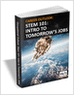MaterialsDesign CenterProcessesUnit ConversionFormulasMathematicsCalculatorsDiscussion ForumTrade PublicationsDirectory Service
STEM Career Outlook

Wages, employment opportunities, and growth projections for STEM jobs.

3D Scanners

A white paper to assist in the evaluation of 3D scanning hardware solutions.

CNC Machining Design Guide

Injection Molding Design Guide

Guide for high quality and cost-effective plastic injection molding.

more free publicationsGlossary » Units » Power (Heat Flow) » Calorie (therm.) Per SecondCalorie (therm.) Per Second (cal (therm.)/s) is a unit in the category of Power (Heat flow). It is also known as calories per second, calorie per second, cal/second, calorie/second. Calorie (therm.) Per Second (cal (therm.)/s) has a dimension of ML2T-3 where M is mass, L is length, and T is time. It can be converted to the corresponding standard SI unit W by multiplying its value by a factor of 4.184.
Note that the seven base dimensions are M (Mass), L (Length), T (Time), Q (Temperature), N (Aamount of Substance), I (Electric Current), and J (Luminous Intensity).

Other units in the category of Power (Heat flow) include Abwatt (emu of Power) (aW), British Thermal Unit (IT) Per Hour (Btu (IT)/h), British Thermal Unit (IT) Per Minute (Btu (IT)/min), British Thermal Unit (IT) Per Second (Btu (IT)/s), British Thermal Unit (therm.) Per Hour (Btu (therm.)/h), British Thermal Unit (therm.) Per Minute (Btu (therm.)/min), British Thermal Unit (therm.) Per Second (Btu (therm.)/s), Calorie (IT) Per Hour (cal (IT)/h), Calorie (IT) Per Minute (cal (IT)/min), Calorie (IT) Per Second (cal (IT)/s), Calorie (therm.) Per Hour (cal (therm.)/h), Calorie (therm.) Per Minute (cal (therm.)/min), Cheval-Vapeur (horsepower) (cv, HP), Dyne-Centimeter Per Second (dyn-cm/s), Erg Per Second (erg/s), Foot-Pound Force Per Hour (ft-lbf/h), Foot-Pound Force Per Minute (ft-lbf/min), Foot-Pound Force Per Second (ft-lbf/s), Foot-Poundal Per Second (ft-pdl/s), Horsepower (550 Ft-Lbf/s) (HP), Horsepower (boiler) (HP), Horsepower (British) (BHP, hp), Horsepower (cheval-Vapeur) (cv, HP), Horsepower (electric) (HP), Horsepower (metric) (HP), Horsepower (water) (HP), Kilogram Force-Meter Per Second (kgf-m/s), Kilowatt (kW), Prony, Ton (refrigeration, UK) (CTR (UK)), Ton (refrigeration, US) (CTR (US)), Volt-Ampere (VA), Watt (W), Watt (int. Mean) (W), and Watt (int. US) (W).N/ARelated PagesSTEM Career Outlook

Wages, employment opportunities, and growth projections for STEM jobs.3D Scanners

A white paper to assist in the evaluation of 3D scanning hardware solutions.CNC Machining Design Guide